Myk's Digital GardenMetastructuralism

#Accessible Intro to Calculus ConceptsAccessible Intro to Calculus Concepts

Hi! If you’re reading this it’s because you want to make sure you understand what Calculus is, and you think you get it (or maybe you don’t and you’re just curious!) and you want to make sure.
I’m not a Math Teacher, but I have had an intuitive grasp of the principles behind calculus since I was a kid and learning to put names to them was exciting to me.

## The Big Picture: What Is Calculus?

Calculus as we generally understand the term is a set of formal methods for reasoning about changing numeric values. Specifically it allows you to do things like graph the slope of a line (Differentiation) or find the area under a curve (Integration). It turns out that these operations are directly translatable into some pretty powerful ways to reason about reality.
Differentiation is the process of shifting focus from “the thing” to “a way the thing is changing with respect to other things”. The result of Differentiation is called the “derivative”, and you actually use this all the time. Next time you’re in your car driving, look at the speedometer. It’s not showing you where you are — it’s showing you how your position is changing with respect to time. Speed is a derivative of location with respect to time.
You use Integration all the time too. Integration says, “Given all of these changes to the thing, what’s the current state of the thing?” The result of Integration is called the “integral” and it represents a new “thing” that is created by “integrating” an initial condition plus a bunch of changes. Think about, for instance, your current bank balance. It’s your initial bank balance (0 for a new account) plus every interaction you’ve ever had all integrated into a single value.
Calculus is a set of axioms and operations that implement these operations, basically, and describe how to perform them against certain kinds of functions (or lines).

## Terms and Definitions

A function, line or curve is a 2-dimensional algebraic function, something like f(x) = 3x - 2x^2 . Crucially, a function must only ever have one y value for a given x value. If it’s a curve that opens to the side, for instance, it’ll have two y values for each x value in most parts and while it may be a line it’s not a function.
The way to read the above function is “Eff of x is three times the value of x minus two times that same value squared.”
What these all have in common is that if you start at x=0 and move to the right or left you can use the value of x to get the exact value of y at that point.
This means that if you take tiny steps (see dx below) and draw a dot at (x, y) for each x, y pair you’ll see that your dots form a line.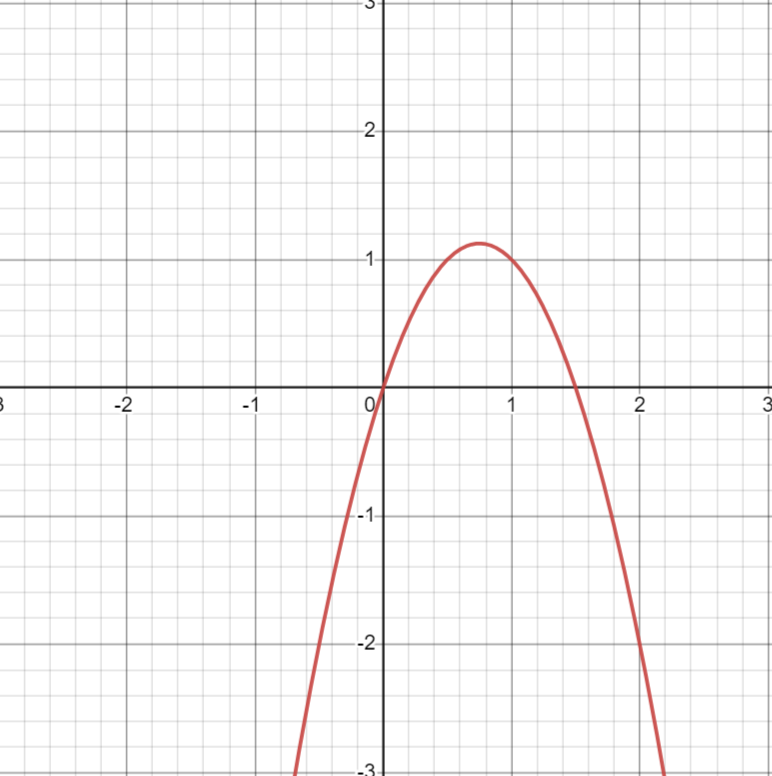f(x) is read “eff of x”. The name of the function is “f” and it takes a single argument, called “x”. We can (and will!) come up with other functions that have other names, but in general y is equal to f(x) — in other words given some value x this function will return the corresponding value of y.
One way to approach reasoning about functions is as follows:
A constant function is one where the value of y is the same regardless of the value of x. An example would be f(x) = 1 — in this line the value of y is always exactly 1 no matter what the value of x is. A constant function is always a horizontal line.
A simple function is one where the value of y is defined in terms of the value of x but those terms are fixed. An example would be f(x) = 2x which says `the value of y is always twice the value of x.’ We need to know the value of x to get y but the relationship between x and y never changes. A simple function is always a straight angled line that intersects with the x-axis exactly once.
A complex function is one where the value of y is defined in terms of the value of x but the relationship between x and y changes as x changes. An example would be f(x) = 3x - 2x^2 above — this means that the relationship between x and y for one value of x is not guaranteed to be the same for another value of x — even a very close second value. A complex function is always a curve.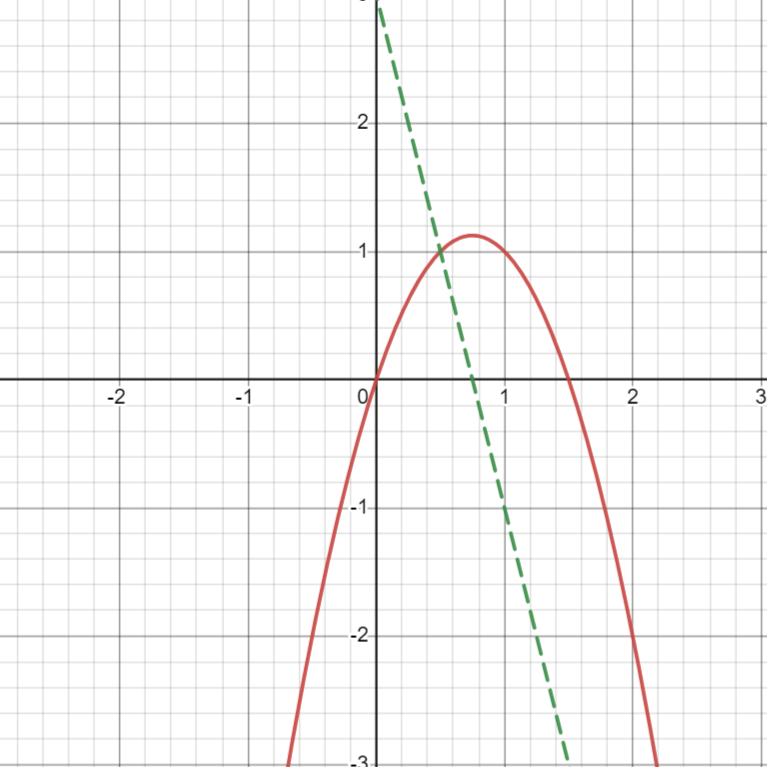The derivative of a function is another line, sometimes called x/dx f(x). Where f(x) gives you the value of y for any given value of x, instead x/dx f(x) gives you the slope of y at the given point along the x-axis; this means that in taking the derivative we lose information, because we can get the slope from a series of y values but we cannot get the y values from a series of slopes.
In the image to the left the derivative is represented as a dotted green line. As you can see, the greater the slope of the red line the higher the derivative’s value, and vice versa.
The point where the curve inflects, or starts moving in the opposite direction, is also the point where (y=0) in the derivative.
The second derivative (graphed here in purple) is the derivative of the derivative of a line. You can see how depending on how complex your initial line is you could have any number of derivatives, each describing the slope at X on the one that came before. A simple function’s derivative is always a constant function, and a constant function’s derivative is always f(x) = 0.
Here our third derivative is graphed in orange — and you can see that, as predicted, our complex function had a simple derivative, which had a constant derivative, which had a derivative of 0.
We cannot take any further derivatives, they will all be 0.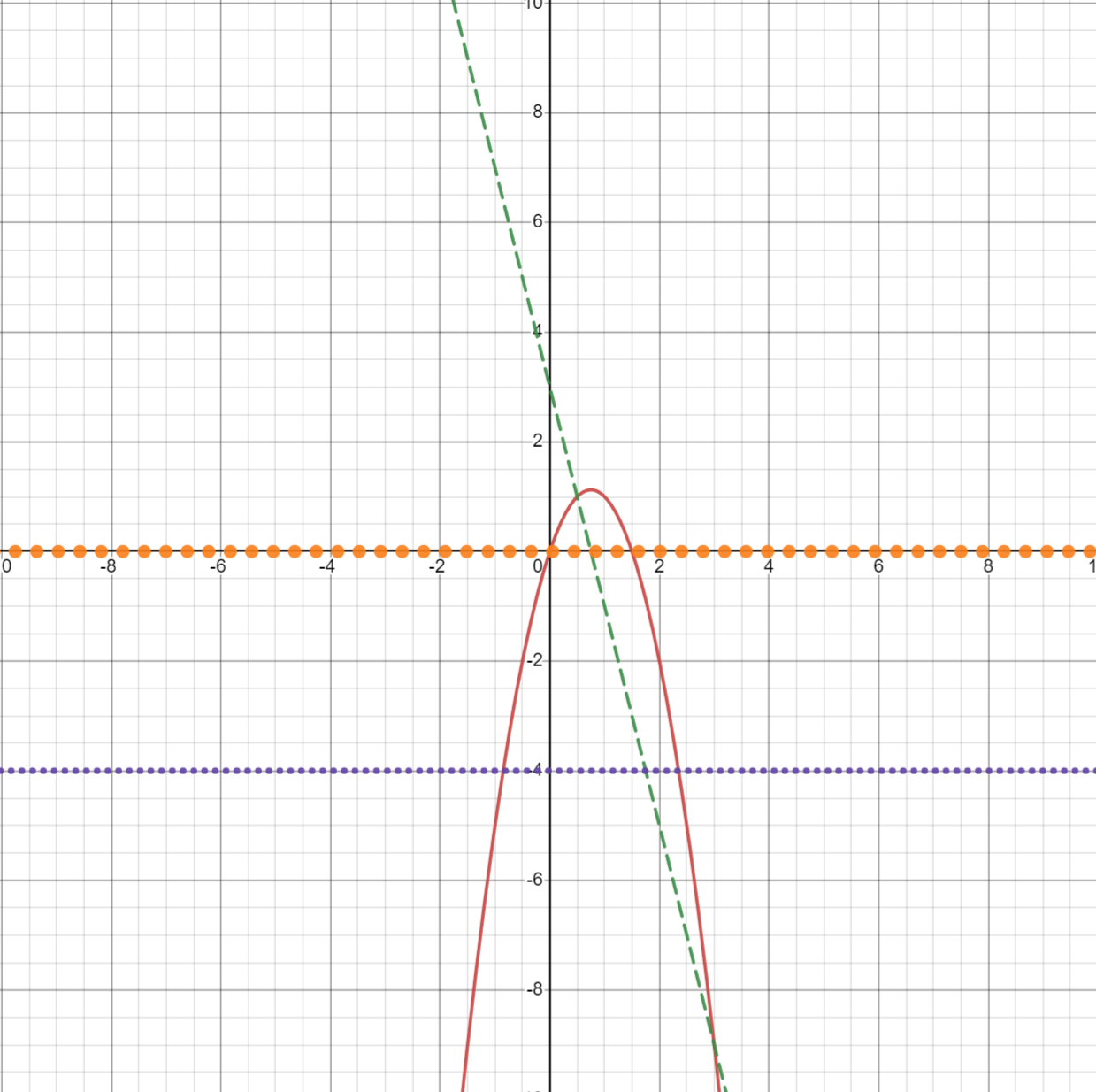The screenshots in this section were taken using the Desmos calculator,
.
dx is important to understand here, we’ll see it again in integrals. dx is the width of the “slice” of the function that you’re looking at. This will make a lot more sense with pictures. In the first picture, we have a dx value that’s large enough that you can see the ways that our approximation is missing information. In the second picture you see the same curve but instead of 8 slices we have 32 slices. This is because the value of dx has decreased by a factor of 4. Imagine how having the ability to reason about “the area of the curve as dx approaches 0”, because we know the width of a slice can’t actually be zero. (Images are screenshots from ).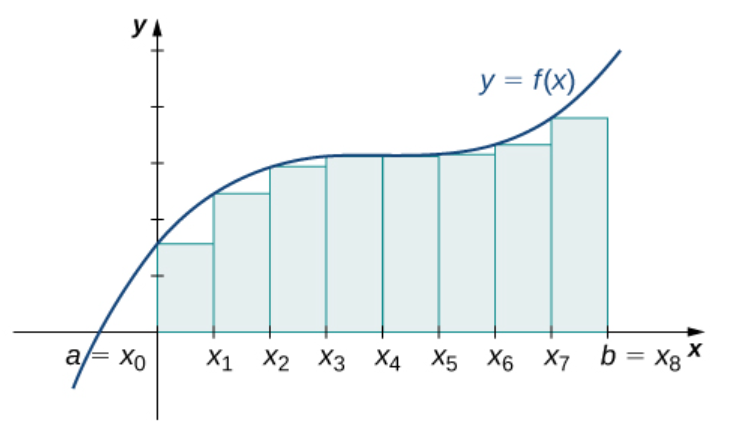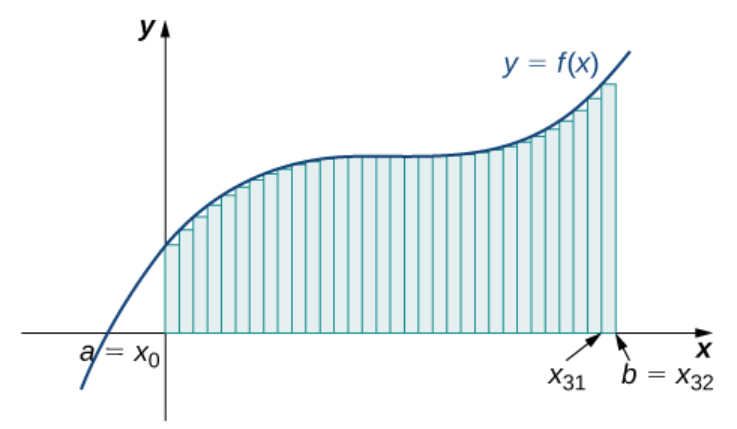A limit is the secret sauce. In calculus, the way we make our rough estimates more correct is to describe them in terms of “limits”. A Limit is a description of what happens to y as x approaches some specific value. This is powerful for a number of reasons, but most importantly it allows us to formally reason about the value of dx as it approaches zero. In other words, a limit is how we specify behavior of a function as dx shrinks down to effectively-but-not-quite zero.
Put another way: limits allow variables to be both zero and not-zero at the same time, and that’s a really useful property.
An integral is the sort of the opposite of the derivative. Instead of reducing our attention to how the slope of the line is changing we expand our attention to include the entire area under the line in a bounded region from a to b.
Writing the mathematical definition for integrals looks like this: ​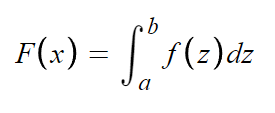Don’t be intimidated. What that’s saying is, let’s define a new function called F that acts on a value called x. For every value of x between a and b, name that value z and apply it to function f (the function you’re taking the integral of) with respect to dx (which in this case we refer to as dz, since x is named z in the inner formula. If this is stressing you out don’t worry about it!)
Still confusing? Think of it this way: it’s a for loop. It’s saying “For every slice under our curve, take the area of that slice (f(z) * dz) and then add all of the resulting areas up.”
How do we know how big the slice is, though? That’s what dz is encoding. dz, or ‘the change in z’, tells you how big our slices are because each slice is one dz away from the previous one. When doing integrals like this the implication is that we are identifying the behavior of the function as dx (or dz in this case) approaches zero.

Taking the area under a constant function is easy — you’re just measuring the area of a rectangle, because y never changes you get a nice straight line to measure under.
If dz here were 2 we would say that this rectangle represented a single slice of width 2.
If dz were .2 we would say that this rectangle represented ten slices, each .2 in width.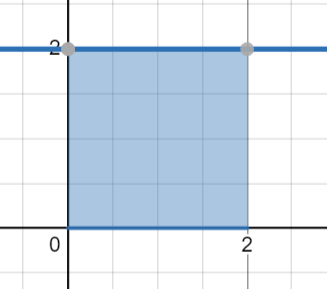Taking the area under a simple function is relatively easy too — you’re finding the area under a triangle. This is a known operation and you don’t need calculus to do it.
If you wanted to though, you could. Here’s the idea: by ‘integrating’ what we’re really doing is drawing thin rectangle whose starting point is some value of x and whose height is y. If those rectangles are too wide, though, there will be gaps between the tops of the rectangles and the line above. So we imagine them as tiny as possible.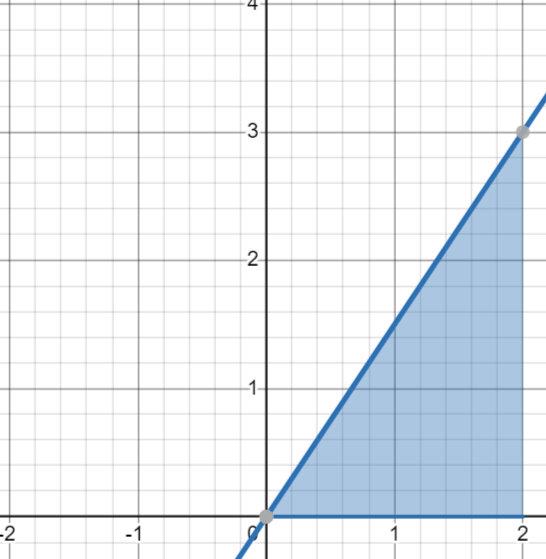This is even more important when taking the area of a complex function, right? One where the space under the line doesn’t conform to a simple geometric shape? In this case calculus is the only way to get this area.
To get the total area “under” this curve we would add up the total blue area and then subtract the total purple area, because it’s “negative area”.
Do you see how we need a tiny dz for this to be accurate?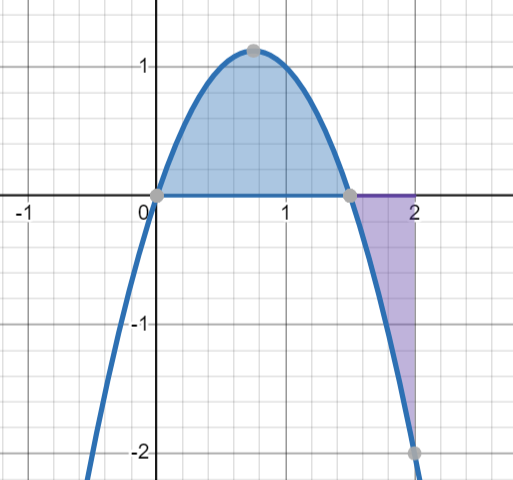### Key Insight

Integration and Differentiation each represent movement along the same axis: information loss in one direction, information gain in the other. Let’s call this line the Information Function. To move towards one pole is to lose specific pieces of information until you reach the inevitable conclusion, 0. To move to the other pole is to constantly add specific pieces of information until you reach the inevitable conclusion, infinity.
A calculus is a set of rules for moving along this Information Function in productive and interesting ways.